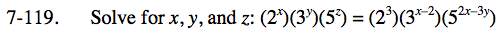Home > INT3 > Chapter 7 > Lesson 7.2.5 > Problem7-119

7-119.

Solve for x, y, and z: (2x)(3y)(5z) = (23)(3x–2)(52x–3y) Homework Help ✎Make a system of equations using the exponents.

x = 3
y = x − 2
z = 2x − 3y

The system of equations gives you the value of x. Substitute the value of x into the second and third equations.
From there, solve as if there were only two equations.